Published: 18 August 2023

# Research on high-speed railway vehicle emergency braking control based on rail surface foreign matter identification

Weihua Wang1
Yaodong Zheng2
Yuanjing Chen3
1Shaanxi College of Communications Technology, Xi’an, Shaanxi, 710018, China
2Xi’an Rail Transit Group Company Limited, Xi’an, Shaanxi, 710016, China
3China Railway Taiyuan Bureau Group Co. Ltd, Taiyuan, Shanxi, 030045, China
Corresponding Author:
Weihua Wang
Article in Press
Views 34

#### Abstract

In order to improve the operation stability of high-speed railway vehicles, an emergency braking control method for high-speed railway vehicles based on the identification of foreign matters on the track surface is proposed. The dynamic frame scanning method is used to build the image acquisition of abnormal objects on the high-speed rail track surface, and the visual sensor and vibration sensor are used to identify and process the features of foreign objects on the high-speed rail track surface. According to the abnormal feature extraction results, the command signal in case of foreign objects is transmitted to the terminal command library of the braking control system. According to the transfer switch of the emergency braking system to display the main emergency disposal output states such as the train emergency disposal information reception, emergency disposal level, emergency disposal type and other information, the joint control method of contact pressure distribution and non-uniform heat flux is adopted, and the joint control method of control system, drive system, disc friction system and signal acquisition system is adopted. The emergency braking control module is established to realize the emergency braking control of high-speed railway vehicles according to the feature recognition results of foreign matters on the rail surface. The test results show that the control module is less affected by the vehicle wheelbase characteristics, track structure characteristics and other factors when using this method for high-speed railway vehicle emergency braking control, and has a strong ability to identify the characteristics of foreign matters on the track surface, which improves the stability and real-time performance of emergency braking.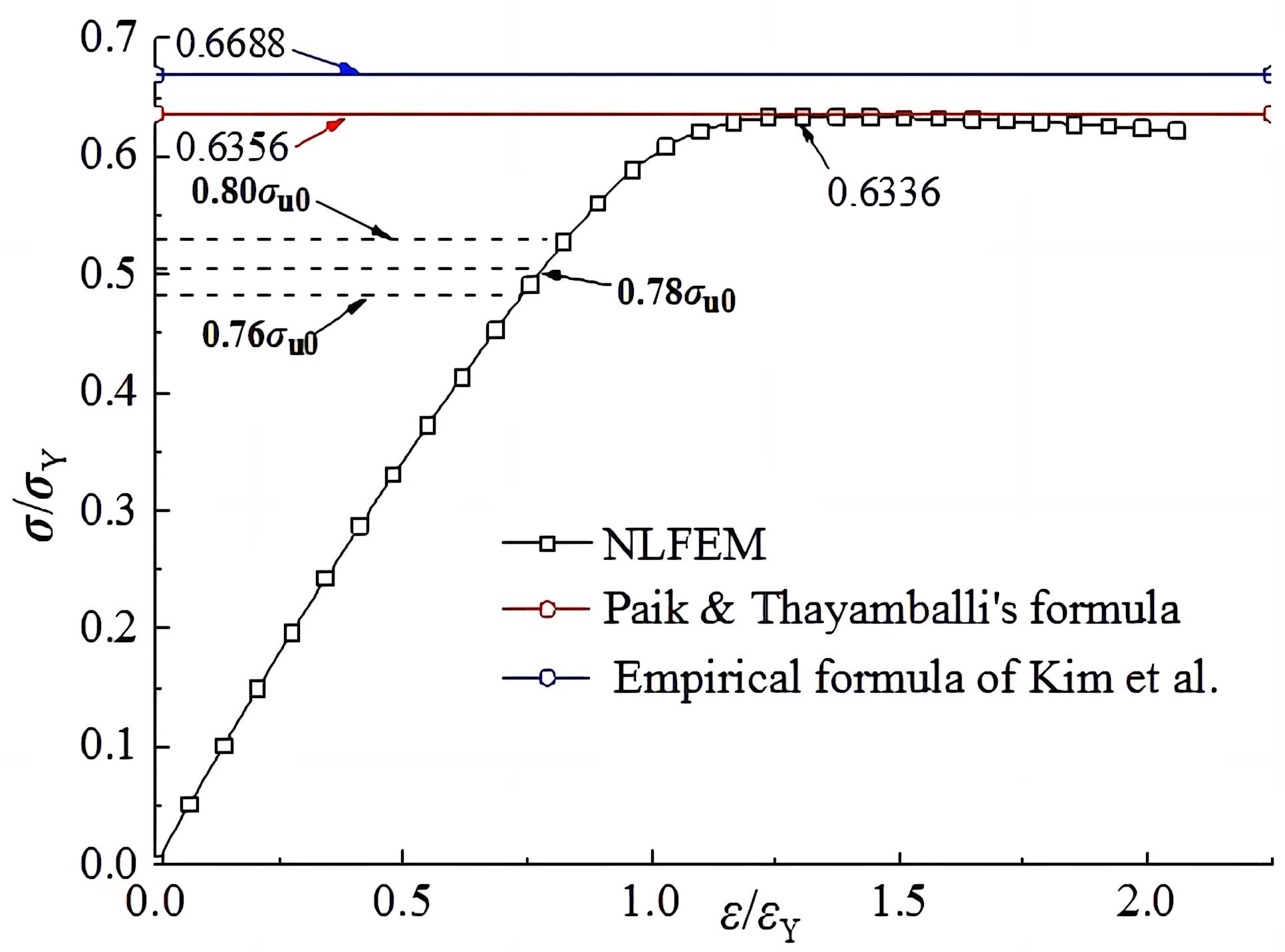#### Highlights

• The dynamic frame scanning method is used to capture images of abnormal objects on the surface of the high-speed rail track. Visual and vibration sensors are employed to identify and process the features of foreign objects on the track.
• Based on the extracted features, command signals are transmitted to the terminal command library of the braking control system to initiate emergency braking for high-speed railway vehicles when foreign objects are detected.
• A joint control method incorporating contact pressure distribution and non-uniform heat flux is utilized, integrating the control system, drive system, disc-disc friction system, and signal acquisition system.
• An emergency braking control module is established to enable emergency braking for high-speed railway vehicles based on the feature recognition results of foreign objects on the rail surface. This enhances braking stability and real-time performance.

## 1. Introduction

High speed trains play a very important role in promoting the development of the national economy, and are also an important means of transportation for people to travel. During the high-speed railway operation, due to the improvement of the operating speed, its transport capacity and transport safety are also concerned by people. During the high-speed railway operation, affected by factors such as track foreign bodies, it is necessary to achieve emergency braking in combination with the distribution of foreign bodies on the track surface. Improve the emergency braking capacity of the train for different operating conditions, so as to make the high-speed railway operation more stable and safe, and the braking effect more efficient. Therefore, research on the emergency braking control method of high-speed railway vehicles based on the identification of foreign matters on the track surface, combined with the detection and identification of abnormal features on the track surface, to improve the stability of the braking control. The related research on emergency braking control methods of high-speed railway vehicles has received great attention .

The emergency braking control of high-speed railway vehicles is based on the analysis of the running performance, running dynamic characteristics and vehicle vibration characteristics of the train. Through the construction of an example railway vehicle model and its vibration test platform, combined with the identification of adaptive suspension system parameters, the active emergency braking control [2, 3] of the train is realized. The adaptive damper is developed in the literature , the damper using intelligent fluid is a semi-active suspension braking method to improve the reliability of control parameters. However, the demand of the system for different operating conditions is limited, which severely limits its practical application. In literature , a method for analyzing and controlling the dynamic characteristics of high-speed train wheels driven by axle box vibration and dynamics model was proposed, and a one-dimensional convolutional neural network analysis model of structure and configuration parameters was established. Through the analysis of the characteristics of mechanical equipment monitoring signals, emergency braking of vehicles was realized, but this method is not good at monitoring environmental processes. In literature , a wheel polygon state recognition and braking control method based on improved EEMD and WVD joint time-frequency analysis is proposed. By collecting the vibration characteristics of vehicle parts of running trains and combining EEMD and WVD joint time-frequency characteristics analysis, the state parameter identification and braking control are realized. However, this method is not robust to the control driven by the mechanical model. Aiming at the above problems, in order to improve the running stability of high-speed railway vehicles, this paper proposes an emergency braking control method for high-speed railway vehicles based on the identification of foreign matters on the track surface. First, according to the abnormal feature extraction results, the command signal in case of foreign matters is transmitted to the terminal command library of the braking control system. Through the joint control method of the control system, the drive system, the disc disc friction system and the signal acquisition system, the emergency braking control module is established to realize the emergency braking control of high-speed railway vehicles according to the feature recognition results of foreign matters on the rail surface. Finally, experimental tests are carried out to show the superior performance of this method in improving the emergency braking control capability of high-speed railway vehicles.

The high-speed railway vehicle emergency braking control method based on track surface impurity recognition has the following innovations: it adopts a dynamic frame scanning method and combines visual sensors and vibration sensors to comprehensively detect and handle abnormal objects on the track surface; by extracting the features of these abnormalities, the braking instruction signals under foreign object conditions are transmitted to the control system to achieve emergency braking control; the emergency braking system toggle switch displays the vehicle’s emergency handling information, allowing the operator to have real-time understanding of the emergency braking situation; the combined control method using contact pressure distribution and non-uniform heat flow improves the stability and real-time performance of emergency braking. In summary, this method innovatively combines multiple aspects such as image acquisition, abnormal object recognition, instruction transmission, and control methods in high-speed railway vehicle emergency braking control, enhancing the stability and effectiveness of emergency braking.

## 2. Analysis and identification of foreign matters on high-speed railway track surface

### 2.1. Analysis on characteristics of foreign matters on high-speed railway track surface

In order to realize the emergency braking control of high-speed rail vehicles based on the identification of foreign matters on the track surface, the dynamic frame scanning method is used to build the image acquisition of abnormal objects on the high-speed rail track surface, build the analysis model of abnormal characteristics of high-speed rail track surface, install the brake pads and sensors of rail vehicles in the high-speed rail braking system , the geometric structure of foreign matter feature extraction on high-speed rail track surface is shown in Fig. 1. By utilizing the dynamic frame scanning method and implementing the necessary hardware components, the proposed approach enables the identification and analysis of surface impurities on the track. This information can then be utilized for effective emergency braking control in high-speed train systems, ensuring the safety and stability of train operations.

Fig. 1Geometric structure of foreign matter feature extraction on high-speed railway track surface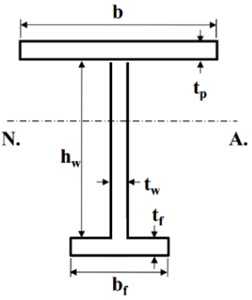On the platform shown in Fig. 1, according to the thermal mechanical coupling damage characteristics analysis of the brake disc, combined with the thermal mechanical coupling response state characteristics detection of the system, the thermal mechanical coupling response state characteristics analysis is carried out according to the common friction block layout of the railway train shown in Fig. 2.

Fig. 2Distribution mode of common friction blocks of high speed railway trains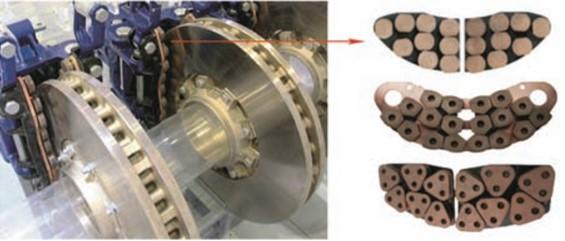The thermal mechanical coupling response state parameters of the obtained brake disc are set as follows.

Initial deflection of brake disc with thermal mechanical coupling damage:

1
${w}_{\mathrm{o}\mathrm{p}\mathrm{l}}={A}_{0}\mathrm{s}\mathrm{i}\mathrm{n}\frac{m\pi x}{l}\mathrm{s}\mathrm{i}\mathrm{n}\frac{\pi y}{b}.$

Brake disc rotation and friction deflection between discs:

2
${w}_{\mathrm{o}\mathrm{c}}={B}_{0}\mathrm{s}\mathrm{i}\mathrm{n}\frac{\pi x}{l}\mathrm{s}\mathrm{i}\mathrm{n}\frac{\pi y}{b}.$

Initial deflection under the constraint of contact pressure distribution and non-uniform heat flux:

3
${w}_{\mathrm{o}\mathrm{s}}={C}_{0}\frac{z}{{h}_{w}}\mathrm{s}\mathrm{i}\mathrm{n}\frac{\pi x}{l}.$

According to the structural model shown in Fig. 2, the spatial distribution characteristics and braking state parameters of the friction block are analyzed, and the load end contraction formula of the control system, drive system, and disc friction system is obtained by combining the characteristic parameter detection of foreign matters on the rail surface  as follows:

4
${\sigma }_{CR1}=\mathrm{\Phi }{\sigma }_{C1}\frac{{A}_{s-n50}+{A}_{pE-n50}}{{A}_{s-n50}+{A}_{p-n50}}.$

Including: $\mathrm{\Phi }$ is an edge function. In order to meet the requirements of high-speed data transmission between the voice synthesis chip and the vehicle terminal, the edge coefficient obtained is equal to:

5
$\mathrm{\Phi }=-1,\mathrm{}\mathrm{}\mathrm{}\mathrm{}\epsilon <-1,$
6
$\mathrm{\Phi }=\epsilon ,-1\le \epsilon \le 1,$
7
$\mathrm{\Phi }=1,\mathrm{}\mathrm{}\mathrm{}\mathrm{}\epsilon >1.$

Among them, ${\sigma }_{C1}$ is the critical stress of the capacitance connected at both ends of the crystal oscillator, in N/mm2, equal to:

8
${\sigma }_{E1}\le \frac{{R}_{eHB}}{2}\epsilon ,{\sigma }_{C1}=\frac{{\sigma }_{E1}}{\epsilon }$
9
${\sigma }_{E1}>\frac{{R}_{eHB}}{2}\epsilon ,{\sigma }_{C1}={R}_{eHB}\left(1-\frac{{R}_{eHB}}{4{\sigma }_{E1}}\epsilon \right).$

Among them, ${R}_{eHB}$ is the minimum equivalent yield stress of high-speed railway vehicle emergency braking based on the identification of foreign matters on the rail surface, in N/mm2, equal to:

10
${R}_{eHB}=\frac{{R}_{\mathrm{e}HB}{A}_{pE1-n50}{l}_{pE}+{R}_{\mathrm{e}Hs}{A}_{s-n50}{l}_{sE}}{{A}_{pE1-n50}{l}_{pE}+{A}_{s-n50}{l}_{sE}}.$

Among them, ${\sigma }_{E1}$ design the appropriate stress for the outside of the terminal housing, in N/mm2, equal to:

11
${\sigma }_{E1}={\pi }^{2}E\frac{{I}_{E-n50}}{{A}_{E-n50}{l}^{2}}{10}^{-4}.$

Among them, ${l}_{pE}$ is the motor adjusted brake disc speed, and ${b}_{E1}$ is the disc friction stress parameter. Therefore, the dynamic frame scanning method is used to build the image acquisition of abnormal objects on the high-speed rail track surface, and visual sensors and vibration sensors are used to identify and process the characteristics of foreign objects on the high-speed rail track surface. According to the abnormal feature extraction results, the command signal in case of foreign matters is transmitted to the terminal command library of the braking control system .

Identification of foreign matters on high-speed railway track surface

Design, process and install brake disc and friction block samples as required, and set ${l}_{sE}$ as the effective width of foreign matters on high-speed rail track surface, and ${b}_{E1}$ as the effective braking distance; ${I}_{E-n50}$ is the moment of inertia under the constraint of rated braking pressure; ${A}_{pE1-n50}$ and ${A}_{pE-n50}$ are the cross section area measured by the width ${b}_{E1}$ and ${b}_{E}$ under the commonly used forged steel material of the train brake disc; ${A}_{E-n50}$ is distribute heat for the friction power between the brake disc and the friction block, ${A}_{s-n50}$ is the cross sectional area of the friction block arrangement of the existing railway train, $\epsilon$ is the relative strain, equal to:

12
$\epsilon =\frac{{\epsilon }_{E}}{{\epsilon }_{Y}}.$

Among them, ${\epsilon }_{E}$ is the unit strain of thermal mechanical coupling response state; ${\epsilon }_{Y}$ is the strain when the unit reaches the yield stress, which slows down the thermal mechanical coupling damage failure of the brake disc equal to:

13
${\epsilon }_{Y}=\frac{{R}_{eHA}}{E}.$

Among them, ${b}_{E1}$ is the effective width of the belt plate corrected by the relative strain, which acts on the rotating motion of the brake disc and the friction between the discs. At this time, the brake control pressure is equal to:

14
${\beta }_{E}>1.0,{b}_{E1}=\frac{s}{{\beta }_{E}},$
15
${\beta }_{E}\le 1.0,{b}_{E1}=s,$

where $s$ is the spacing between ribs, and the brake disc and friction block are set to face to face contact configuration . At this time, the high-speed rail track surface foreign matter feature recognition output:

16
${\beta }_{E}>1.25,{b}_{E}=\frac{2.25}{{\beta }_{E}}-\frac{1.25}{{\beta }_{E}^{2}},$
17
${\beta }_{E}\le 1.25,{b}_{E}=s.$

According to the feature recognition results, the single rib model and the three rib model shown in Fig. 3 are used to realize the simplified disc brake control analysis of the friction block during the emergency braking of the vehicle.

Fig. 3Example of simplified disc brake control of friction block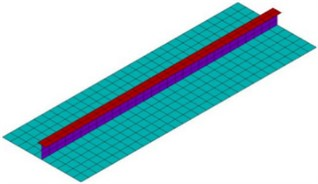a) Single reinforcement model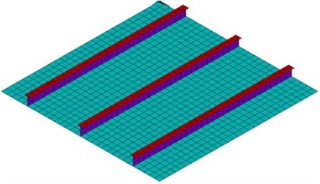b) Three bar model

## 3. Realization of emergency braking control algorithm

### 3.1. Parameter optimization of emergency braking disposal output state model

Make full use of all kinds of prior information to improve the estimation accuracy, build an emergency braking disposal output state model parameter optimization model [11-13], conduct reliability evaluation according to the prior data, and obtain the buckling load end contraction formula of the braking control unit as follows:

18
${\sigma }_{CR3}=\mathrm{\Phi }\frac{{10}^{3}{b}_{E}{t}_{n50}{R}_{eHp}+\left({h}_{we}{t}_{w-n50}+{b}_{f}{t}_{f-n50}\right){R}_{eHs}}{{10}^{3}s{t}_{n50}+{h}_{w}{t}_{w-n50}+{b}_{f}{t}_{f-n50}},$

where: ${b}_{E}$ is the population with random variable taken as life; ${H}_{we}$ is the effective height of the stiffened plate web brake control unit, in mm, equal to:

19
${\beta }_{w}<1.25,{h}_{we}=\left(\frac{2.25}{{\beta }_{w}}-\frac{1.25}{{\beta }_{w}^{2}}\right){h}_{w},$
20
${\beta }_{w}<1.25,{h}_{w}={h}_{we}.$

Among them, ${\beta }_{w}$ is the total number of abdominal failures, equal to:

21
${\beta }_{w}=\frac{{h}_{w}}{{t}_{w-n50}}\sqrt{\frac{\epsilon {R}_{eHs}}{E}}.$

Then the fatigue damage factor ${D}_{sp}$ of high-speed railway vehicle emergency braking can be defined as:

22
${D}_{sp}=\frac{{A}_{c}}{A},$

wherein, ${A}_{c}$ is the damage area set in the face to face contact configuration between the brake disc and the friction block, and $A$ is the damping force generated by the nylon cylinder system. Analyze the working mode of the shock absorber and obtain the residual ultimate strength:

23
$\frac{{\sigma }_{uc}}{{\sigma }_{u}}=\frac{{A}_{e}}{A},$

where ${\sigma }_{u}$ and ${\sigma }_{uc}$ respectively represents the limit value of the magnetic field strength varying with the current. ${A}_{e}$ represents the large bearing area of the damping force in the damping cylinder system, and its value is equal to:

24
${A}_{e}=A-{A}_{c}.$

Substitute Eq. (24) into Eq. (23) to get:

25
${\sigma }_{uc}={\sigma }_{u}\left(1-{D}_{sp}\right).$

According to the main emergency disposal output status  such as train emergency disposal information reception, emergency disposal level, emergency disposal type and other information displayed by the change-over switch of the emergency braking system, the emergency braking control design is carried out using the joint control method of contact pressure distribution and non-uniform heat flux.

### 3.2. Transmission of braking control command and anti-interference processing

Through the joint control method of control system, drive system, disc friction system and signal acquisition system, the emergency braking control module is established, and the emergency braking control  for high-speed railway vehicles is realized according to the feature recognition results of foreign matters on the rail surface, and the magnetorheological effect shows an exponential function relationship:

26
$\left\{\begin{array}{l}\frac{{c}_{p}}{b}=f\left(N\right)={a}_{1}{e}^{{b}_{1}N}+{c}_{1},\\ \frac{{c}_{s}}{{h}_{w}}=f\left(N\right)={a}_{2}{e}^{{b}_{2}N}+{c}_{2}.\end{array}\right\$

By substituting Eq. (26), it is analyzed that the relationship between the ultimate strength of VS-MR damper spring and the number of cycles is also an exponential function:

27
$\frac{{\sigma }_{u}}{{\sigma }_{Y}}=\frac{{a}_{3}{e}^{{b}_{3}N}+{c}_{3}}{\sqrt{0.995+0.936{\lambda }^{2}+0.170{\beta }^{2}+0.188{\lambda }^{2}{\beta }^{2}-0.067{\lambda }^{4}}}.$

Since the stress strain curve of the braking control system is measured by MTS fatigue testing machine, the braking stress strain function is:

28
$\epsilon =\mathrm{l}\mathrm{n}\left(1+e\right),$
29
$\sigma =S\left(1+e\right),$

where $S$ and $e$ are engineering stress and engineering strain respectively; $\sigma$, $\epsilon$ are true stress and true strain. The magnetic fields generated around the energized coil include:

30
$\frac{\epsilon }{{\epsilon }_{\mathrm{Y}}}=\frac{\sigma }{{\sigma }_{\mathrm{Y}}}+K\text{'}{\left(\frac{\sigma }{{\sigma }_{Y}}\right)}^{n\text{'}}.$

Among where $\sigma$ and $\epsilon$ represent the true stress and true strain of braking control force respectively; ${\epsilon }_{Y}={\sigma }_{Y}/E$ is the yield strain, then:

31
$\epsilon =\frac{\sigma }{E}+K\text{'}\frac{\sigma }{E}{\left(\frac{\sigma }{{\sigma }_{Y}}\right)}^{{n}^{\text{'}}-1}.$

Cyclic stress control index based on Ramberg Osgood model ${n}^{\text{'}}=$5.14. To sum up the above analysis, an emergency braking control module is established to realize the emergency braking control of high-speed railway vehicles according to the feature recognition results of foreign matters on the track surface, and the emergency braking control of vehicles is realized according to the change of MTS servo hydraulic pressure controlled by the computer.

## 4. Experimental test

In the experiment, the designed high-speed railway vehicle emergency braking control system uses VS-MR controller to analyze the change of equivalent stiffness coefficient with current. When the coil current changes from О increasing to 0.9 A, the equivalent stiffness coefficient increases from 14.651 kN/m to 41.580 kN/m, and the equivalent stiffness coefficient increases by 2.84 times. The experiment utilized LabVIEW software for the operation and data recording of the automotive brake test system. LabVIEW is a powerful graphical development environment that allows for the quick setup of experimental systems, as well as data acquisition, processing, and analysis. With the interface provided by LabVIEW, it is convenient to input the excitation displacement data and establish real-time communication with the NICompactRio controller. The working principle of the vehicle braking test system is as follows: write the excitation displacement data in computer 1, read the excitation data through LabVIEW software and transmit the data to the NI CompactRio controller, and the reading frequency of the excitation signal is 100 Hz; Then the NICompactRio controller controls the movement of six servo electric cylinders. As the current applied to the designed VS-MR damper increases, the change of its equivalent stiffness coefficient is approximately positive linear. The distribution model of four kinds of rail surface foreign matters is shown in Fig. 4.

According to the above test parameter settings, the comparison of control parameters under various braking control modes (unit: MPa) is analyzed as shown in Table 1.

Fig. 4Distribution model of four kinds of foreign matters on rail surface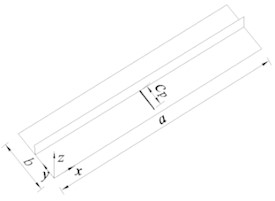a) Model-AP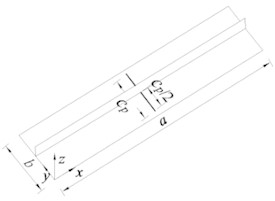b) Model-SP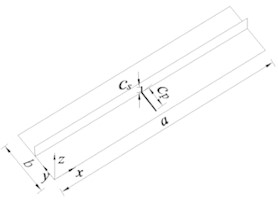c) Model-AB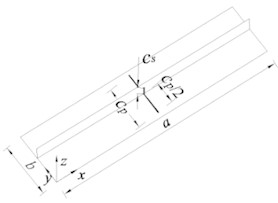d) Model-SB

Table 1Comparison of control parameters under various braking control modes

 Flexion pattern(Model) Load Simulation value(Single reinforcement) Simulation value(Three bars) SimplifyFormula value Maximumerror Brake controlled buckling S1 Monotonic load 336.816 358.243 372.652 –9.62 % Cyclic correction 313.772 327.063 338.414 –7.28 % Cyclic correction+Stress damage 293.815 304.568 311.949 –5.81 % Brake control roll buckling S2 Monotonic load 339.179 370.202 383.827 –11.63 % Cyclic correction 319.075 325.534 343.393 –7.08 % Cyclic correction+fatigue damage 294.119 300.118 316.536 –7.37 % Braking control local buckling S3 Monotonic load 392.560 405.166 405.715 –2.03 % Cyclic correction 321.849 331.268 356.832 –9.8 % Cyclic correction+Stress damage 293.972 305.362 326.416 –9.94 %

According to the analysis of the above numerical simulation results, the emergency braking control can improve the vehicle's ability to identify the state characteristics of foreign matters, and the braking control effect is good. The identification results of foreign matters on the test track surface are shown in Fig. 5, and the finite element analysis results of braking control force under different test pieces are shown in Fig. 6.

According to the analysis of Fig. 6, the method in this paper achieves emergency braking of high-speed railway vehicles and has good ability to identify foreign matters on the track surface. The control performance curves of different methods are tested and the average stress average strain curves are obtained as shown in Fig. 7. According to the analysis of Fig. 7, the axial load axial displacement curves of test pieces P5, STP2 and STP4 under cyclic load. Due to the local contact and clearance between the test piece and the upper and lower tooling, as well as the influence of bolts, gaskets and other components, the readings of the dial indicators symmetrically installed on both sides during the measurement process are not completely consistent during the tension and compression loading test, resulting in a certain error in displacement calculation, which improves the brake control response capability.

Fig. 5Identification results of foreign bodies on rail surface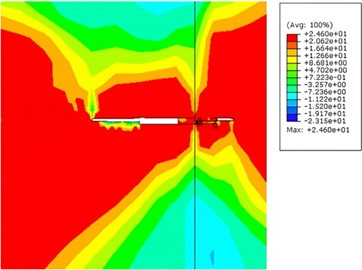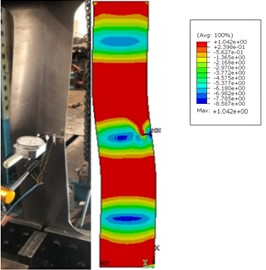Fig. 6Finite element analysis results of brake control force under the same test piece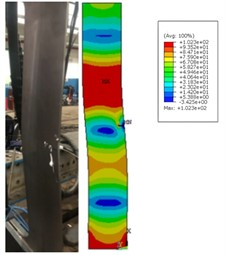a) Test piece P0 (uniaxial compression)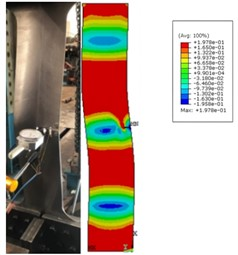b) Test piece P5 collapses after cycling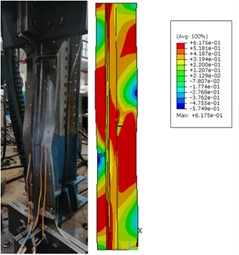c) Test piece STP0 (uniaxial compression)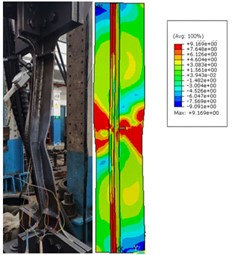d) Test piece STP4 collapses after cycling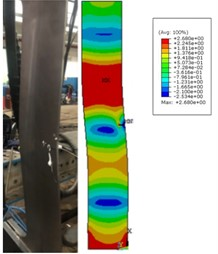e) Test piece P0 (uniaxial compression)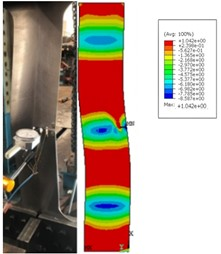f) Test piece P5 collapses after cycling

Fig. 7Average stress average strain curve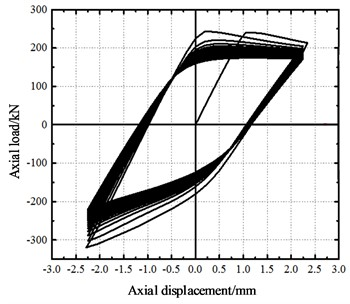a) STP2 simulation of test piece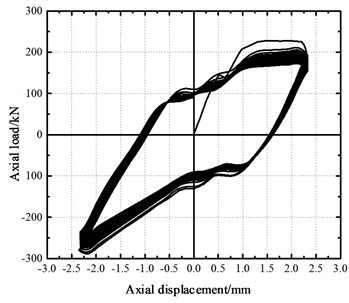b) Test piece STP2 test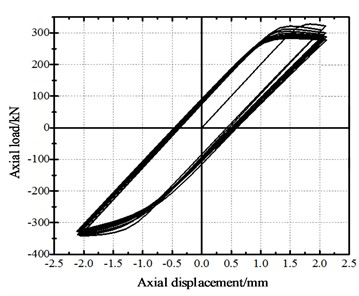c) STP4 simulation of test piece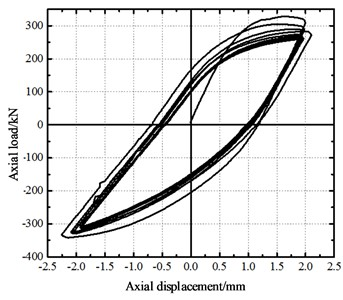d) Test piece STP4 test

As shown in Fig. 8. The analysis and simulation results show that the control module is less affected by the vehicle wheelbase characteristics, track structure characteristics and other factors when the method is used for high-speed rail vehicle emergency braking control, and has a strong ability to identify the characteristics of foreign matters on the track surface, which improves the stability and real-time performance of emergency braking.

Fig. 8Control performance curve object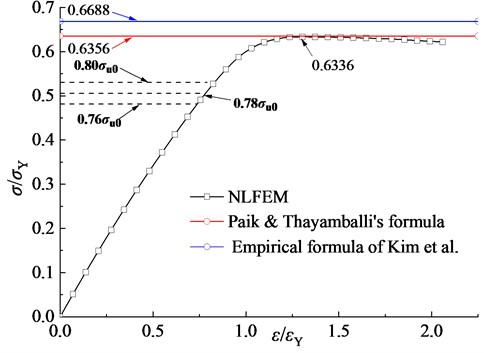To further validate the superiority of the emergency braking method based on the recognition of surface impurities on the track, we conducted comparative experiments using the methods described in reference  and reference . The test indicators chosen were braking distance, braking time, average deceleration, and success rate of recognition. The experimental results are presented in Table 2.

Table 2Comparison of experimental results for emergency braking methods

 Experimental methods Evaluating indicator Braking distance (m) Braking time (s) Average deceleration (m/s2) Foreign object recognition success rate (%) Reference  Method 120 4 3 58 Reference  Method 115 3.5 3.2 60 Proposed method 90 1.5 5 85

Based on the experimental results from Table 2, it can be observed that the proposed method outperforms the other two methods in terms of braking distance, braking time, average deceleration, and success rate of recognition. The braking distance of the proposed method is significantly reduced to 90 meters, which is superior to the distances achieved by the other methods. The proposed method also demonstrates a faster braking time of just 1.5 seconds, compared to the other two methods. Additionally, the average deceleration of the proposed method is the highest at 5 m/s2. Furthermore, the proposed method achieves the highest success rate of 85 % in impurity recognition.

## 5. Conclusions

Incorporating the distribution of surface impurities on the high-speed rail, the goal is to implement emergency braking and enhance the emergency braking capability of trains under different operating conditions. This aims to ensure a smoother and safer operation of the high-speed rail with more efficient braking effects. A method for emergency braking control of high-speed trains based on the recognition of surface impurities on the track is proposed. The objective of this method is to establish a parameter optimization model to improve the accuracy of impurity recognition and the output state model of emergency braking disposal. Moreover, the display of the emergency braking system's conversion switch includes important status information such as receiving emergency disposal information, emergency disposal level, and emergency disposal type.

To achieve emergency braking control, a combined control method involving contact pressure distribution and non-uniform heat flow is used. This design takes into account the operating environment of high-speed trains and provides an effective emergency braking solution. Through this combined control method, better response to emergency situations is achieved, ensuring more efficient braking effects on the train. After conducting a series of experimental studies, the following conclusions have been drawn. Firstly, the proposed emergency braking control method based on the recognition of surface impurities on the track shows good performance in emergency braking control for high-speed railway vehicles. Secondly, due to minimal influence from factors such as vehicle axle distance characteristics and track structures, the method demonstrates strong adaptability. Experimental results indicate that the method is capable of effectively identifying surface impurity features on the track with a success rate of 85 %. Lastly, the method enhances the stability and real-time response of emergency braking, effectively improving the smoothness and safety of high-speed train operation.

In summary, the high-speed train emergency braking control method based on the recognition of surface impurities on the track achieves significant results by fully utilizing prior information and employing a combined control method involving contact pressure distribution and non-uniform heat flow during high-speed rail operations. The practicality and application value of this method have been demonstrated through the research findings, providing a feasible solution for emergency braking control in high-speed trains.

30 May 2023
Accepted
21 July 2023
Published
18 August 2023
SUBJECTS
Vibration in transportation engineering
Keywords
rail surface foreign matter recognition
high-speed rail
vehicle
emergency braking
control
vibration sensor
Acknowledgements

The authors have not disclosed any funding.

Data Availability

All datasets generated for this study are included within the article.

Author Contributions

Weihua Wang: Writing – original draft preparation, project administration. Yaodong Zheng: Writing – review and editing, supervision. Yuanjing Chen: data curation, investigation, validation, visualization.

Conflict of interest

The authors declare that they have no conflict of interest.